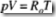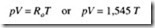# CHARACTERISTICS OF COMPRESSED AIR:DALTON’S LAW

DALTON’S LAW

This states that the total pressure of a mixture of ideal gases is equal to the sum of the partial pressures of the constituent gases. The partial pressure is defined as the pres­ sure each gas would exert if it alone occupied the volume of the mixture at the mix­ ture’s temperature.

Dalton’s law has been proved experimentally to be somewhat inaccurate, the total pressure often being higher than the sum of the partial pressures. This is especially true during transitions as pressure is increased. However, for engineering purposes it is the best rule available and the error is minor.

When all contributing gases are at the same volume and temperature, Dalton’s law can be expressed as

p=pa+Pb+Pc+ …

AMAGAT’S LAW

This is similar to Dalton’s law, but states that the volume of a mixture of ideal gases is equal to the sum of the partial volumes that the constituent gases would occupy if each existed alone at the total pressure and temperature of the mixture. As a formula this becomes:

PERFECT GAS FORMULA

Starting with Charles’ and Boyle’s laws, it is possible to develop the formula for a given weight of gas:

where W is weight and R 1 is a specific constant for the gas involved. This is the perfect gas equation. Going one step further, by making W,in pounds, equal to the molecular weight of the gas (1 mole), the formula becomes:In this very useful form, R is known as the universal gas constant, has a value of 1,545, and is the same for all gases. The specific gas constant (R 1 for any gas can be obtained by dividing 1,545 by the molecular weight. R is only equal to 1,545 when gas pressure (p) is in psia; volume (V) is expressed as cubic feet per pound mole; and temperature (T) is in Rankin or absolute, i.e., ‘F + 460.

Avogadro’s law states that equal volumes of all gases, under the same conditions of pressure and temperature, contain the same number of molecules. This law is very important and is applied in many compressor calculations.

The mole is particularly useful when working with gas mixtures. It is based on Avogadro’s law that equal volumes of gases at given pressure and temperature (pT) conditions contain equal number of molecules. Because this is so, the weight of these equal volumes will be proportional to their molecular weights. The vol­ ume of one mole at any desired condition can be found by the use of the perfect gas law.GAS AND VAPOR

By definition, a gas is that fluid form of a substance in which it can expand indefi­nitely and completely fill its container. A vapor is a gasified liquid or solid or a sub­ stance in gaseous form. These definitions are in general use today.

All gases can be liquefied under suitable pressure and temperature conditions and therefore could also be called vapors. The term gas is most generally used when con­ ditions are such that a return to the liquid state, i.e., condensation, would be difficult within the scope of the operations being conducted. However, a gas under such condi­ tions is actually a superheated vapor.

CHANGES OF STATE

Any given pure substance may exist in three states: as solid, as liquid, or as vapor. Under certain conditions, it may exist as a combination of any two phases, and changes in conditions may alter the proportions of the two phases. There is also a con­ dition where all three phases may exist at the same time. This is known as the triple point. Water has a triple point at near 320oF and 14.696 psia. Carbon dioxide may exist as a vapor, a liquid, and a solid simultaneously at about -69.6oF and 75 psia. Under proper conditions, substances may pass directly from a solid to a vapor phase. This is known as sublimation.

Changes of State and Vapor Pressure

As liquid physically changes into a gas, its molecules travel with greater velocity and some break out of the liquid to form a vapor above the liquid. These molecules create a vapor pressure that, at a specified temperature, is the only pressure at which a pure liquid and its vapor can exist in equilibrium. If in a closed liquid-vapor system the volume is reduced at constant temperature, the pressure will increase imperceptibly until condensation of part of the vapor into liquid has lowered the pressure to the original vapor pressure corresponding to the temperature. Conversely, increasing the volume at constant temper­ ature will reduce the pressure imperceptibly and molecules will move from the liquid phase to the vapor phase until the original vapor pressure has been restored. For every substance, there is a definite vapor pressure corresponding to each temperature.

The temperature corresponding to any given vapor pressure is obviously the boiling point of the liquid and also the dew point of the vapor. Addition of heat will cause the liquid to boil and removal of heat will start condensation. The three terms-saturation temperature, boiling point, and dew point-all indicate the same physical temperature at a given vapor pressure. Their use depends on the context in which they appear.

Critical Gas Conditions

There is one temperature above which a gas cannot be liquefied by pressure increase. This point is called the critical temperature. The pressure required to compress and condense a gas at this critical temperature is called the critical pressure.